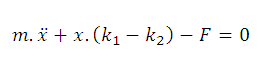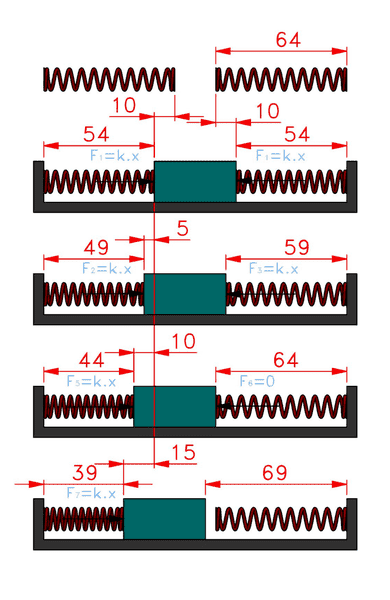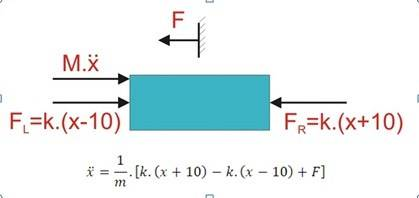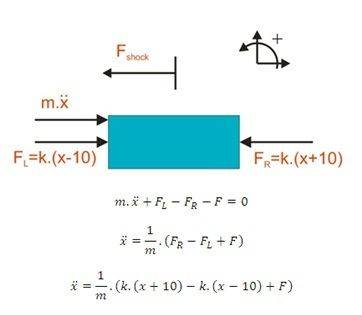# Calculation of the mass springs system

I want to simulate such a system in Simulink.
It is a system of mass between two compression, spring preload. Springs are compressed length of 64 mm to 54 mm. Acts on mass shock 50G for 10ms. The coefficient of spring constant is changed depending on the position and direction of movement mass.
But I can not deal with the equation.
So far I only have such an equation, but probably not correct.how to calculate such a system?OldEngr63
Gold Member
You have the wrong differential equation. Simulink will be of no use until you get the correct DE.

Draw a FBD for the mass and apply Newtons 2nd law.

Hi, I have a thought that I have a bad differential equation. I do not know how to start, how do I calculate such a system, see. my picture.
Because if there were extension springs, it would be easy.
However, if used compression springs, so that's then I do not calculate.

OldEngr63
Gold Member
First, a question: Are these springs attached to the block (so that they can apply tension as well as compression), or are they compression only?

If they apply compression only, then when the displacement of the block exceeds the initial compression, one of the springs will exert zero force. If they are attached to the block, then they can apply tension forces when the displacement would otherwise disengage them.

Start from the beginning by writing the force in each springs as a function of the position of the block. Then write Newton's 2nd law (Sum F = m*a) to get the differential equation.

(Sorry about the type face problems; the system has a mind of its own.)

OldEngr63
Gold Member
I don't think you have ever said so, but I presume that the two springs are identical, and you did indicate that they operate in compression only. Your top figure shows each spring with a free length of 64 (mm?) and a equilibrium compressed length of 54 mm. Thus the initial compressive force in each spring is k*(10) = 10 k. Consider x to be measured to the right from the equilibrium position of the block. The spring forces are
For left spring ---
FL = k (x-10) for x <= 10
and
FL = 0 for x > 10
For right spring ---
FR = k (x+10) for x >= -10
and
FR = 0 for x < -10
Then the equation of motion is
m*(d2x/d2t) = FL - FR
where FL and FR are given by the piecewise descriptions above.

Notice that for abs(x)<=10, the stiffness is 2*k, for for abs(x)>10 the stiffness drops to simply k.

The springs are not permanently connected with the mass, see video
Are the compression springs.
Yes if the mass goes in one direction, a situation arises when one spring would not push the mass, and then vice versa, tells more about the video.
I sorry for having not know good English.

video is obviously abbreviated, thus mass will move until it reaches equilibrium, the initial position. for example. friction

OldEngr63
Gold Member
video is obviously abbreviated, thus mass will move until it reaches equilibrium, the initial position. for example. friction

Sorry, I do not understand what you wrote. You think this way?OldEngr63
Gold Member
To begin with, take x, d2x/d2t, and the force sum positive in the same direction (positive to the right is the usual convention).

Notice, however, that the spring forces can only be described piecewise, not by a single expression.

Notice also that there is no M.d2x/d2t force, so this should not appear in the FBD. Also, I don't recall there being an F in the original problem statement (no subscript), so this needs to be removed from the FBD as well (unless I have overlooked something).

The equation of motion is, as I wrote it above,
m*(d2x/d2t) = FL - FR

So once again. Because I'm not even sure if I understand you.
Mass is between two compression springs. Force is applied on the system about the size of 50G for 10ms.
So the way it is right now?OldEngr63
Gold Member
You seem to be quite confused on what Newton's Second Law says (have you been drinking d'Alembert?). Newton's Second Law says
M.a = sum of forces
What you have written looks like
M.a + sum of forces = 0 (that confusing d'Alembert stuff!, stay away from it!!)
Do you see the difference?

Your FBD should not include an arrow labeled m.x-dot-dot. This is not a force!! Only show forces on the FBD.

If you think m.x-dot-dot is a force, ask yourself where the reaction forces is. The third law says that for every force (action) there is an equal and opposite force (action) somewhere in the universe. Where is the reaction to the m.x-dot-dot term? There is not any, not to be found anywhere in the cosmos!!

Also, you have written the forces in the form that is valid only for abs(x)<=10. This cannot be strictly correct because the forces require the piecewise definition I gave earlier.

OldEngr63
Gold Member
Look at your equation and ask yourself, "How can this apply to the 4th figure down where the right-side spring has lost contact with the block?"

It is true, the fourth image above equations will not apply because we get a negative value. Then what next?

hi, can not someone help me? I will be grateful for any advice.